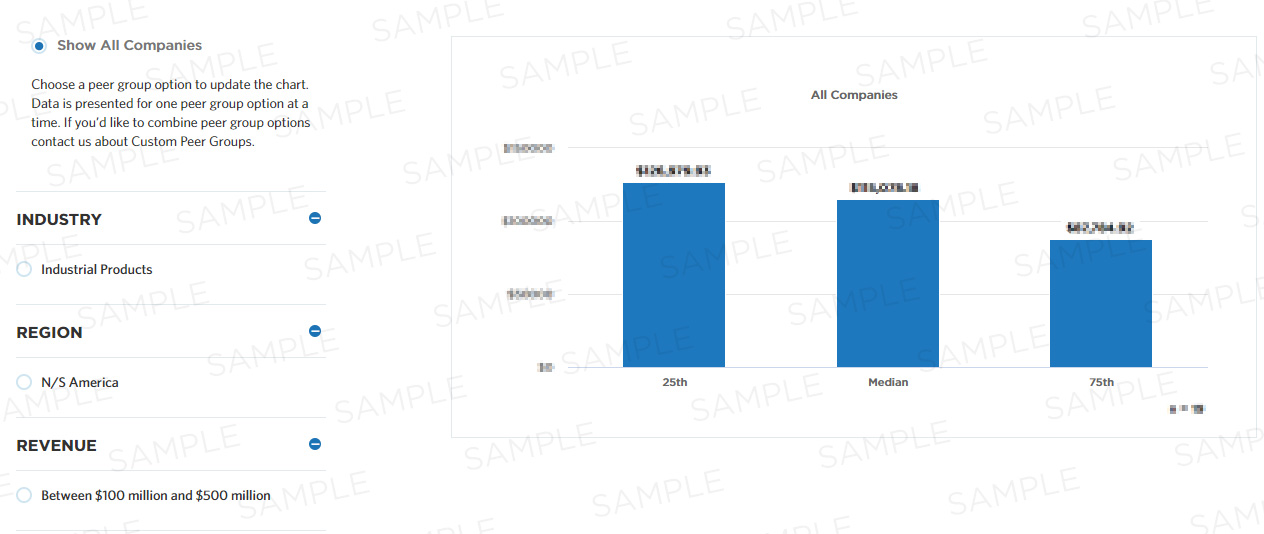# Inventory accuracy

This measure calculates average inventory accuracy, which is the absolute value of the sum of the variance between physical inventory and perpetual inventory (i.e. inventory that is accounted for on a continuous basis as opposed to discrete points in time). It is part of a set of Process Efficiency measures that help companies optimize the performance of their "operate warehousing" process by minimizing waste and refining resource consumption.

## Benchmark Data

Measure Category:
Process Efficiency
Measure ID:
100781
Total Sample Size:
3,687 All Companies
Performers:
25th
-
Median
-
75th
-
Key Performance
Indicator:
Yes## Compute this Measure

Units for this measure are percent.

`Inventory accuracy`

## Key Terms

#### Process efficiency

Process efficiency represents how well a process converts its inputs into outputs. A process that converts 100% of the inputs into outputs without waste is more efficient than one that converts a similar amount of inputs into fewer outputs.

#### Median

The metric value which represents the 50th percentile of a peer group. This could also be communicated as the metric value where half of the peer group sample shows lower performance than the expressed metric value or half of the peer group sample shows higher performance than the expressed metric value.